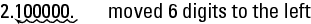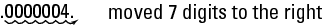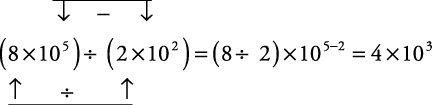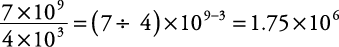## Scientific Notation

Very large or very small numbers are sometimes written in scientific notation. A number written in scientific notation is a decimal number between 1 and 10 multiplied by a power of 10.

##### Example 1

Express the following in scientific notation.

1. 2,100,000 written in scientific notation is 2.1 × 10 6. Simply place the decimal point to get a number between 1 and 10 and then count the digits to the right of the decimal to get the power of 10.2. 0.0000004 written in scientific notation is 4.0 × 10 –7. Simply place the decimal point to get a number between 1 and 10 and then count the digits from the original decimal point to the new one.Notice that number values greater than 1 have positive exponents as the power of 10 and that number values between 0 and 1 have negative exponents as the power of 10.

#### Multiplication in scientific notation

To multiply numbers in scientific notation, multiply the numbers that are between 1 and 10 together to get a whole number. Then add the exponents on the 10's to get a new exponent on 10. It may be necessary to make adjustments to this answer in order to correctly express it in scientific notation.

##### Example 2

Multiply and express the answers in scientific notation.

1.2.This answer must be changed to scientific notation (first number from 1 to 9):

30 × 10 12 = 3.0 × 10 1 × 10 12 = 3.0 × 10 13

1. c#### Division in scientific notation

To divide numbers in scientific notation, divide the numbers that are between 1 and 10 to get a decimal number. Then subtract the exponents on the 10s to get a new exponent on 10. It may be necessary to make adjustments to this answer in order to correctly express it in scientific notation.

##### Example 3

Divide and express the answers in scientific notation.

1.2.3.4.This answer must be changed to scientific notation.

0.4 × 10 2 = 4 × 10 –1 × 10 2 = 4 × 10 1

1. e